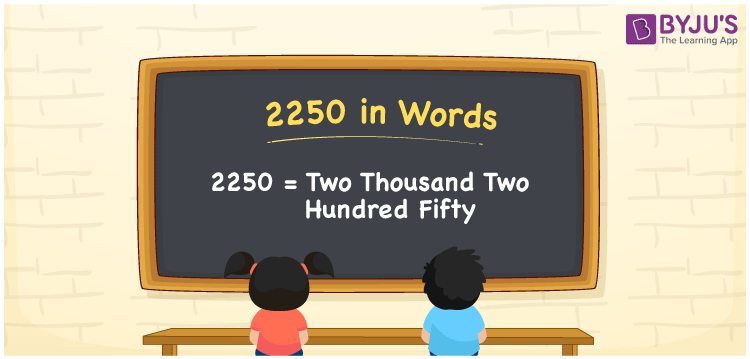# 2250 in Words

We can write 2250 in words as Two thousand two hundred fifty. Suppose you bought a wall clock that costs Rs. 2250, you can say, “I purchased a wall clock for Two thousand two hundred fifty rupees”. Similarly, we can employ the word form of the cardinal number 2250 in general conversations.

 2250 in words Two thousand two hundred fifty Two thousand two hundred fifty in Numbers 2250

## 2250 in English Words

Using the English alphabet, we can write numbers in words. Thus, we can spell 2250 in words as “Two thousand two hundred fifty.## How to Write 2250 in Words?

Place value chart is an effective tool that can be used to convert the number 2250 into words. This can be done as follows.

 Thousands Hundreds Tens Ones 2 2 5 0

Here, ones = 0, tens = 5, hundreds = 2, and thousands = 2.

Thus, we can write the expanded form as:

2 × Thousand + 2 × Hundred + 5 × Ten + 0 × One

= 2 × 1000 + 2 × 100 + 5 × 10 + 0 × 1

= 2000 + 200 + 50

= Two thousand + Two hundred + Fifty

= Two thousand two hundred fifty

Hence, 2250 in words = Two thousand two hundred fifty.

2250 is a natural number that precedes 2251 and succeeds 2249.

2250 in words – Two thousand two hundred fifty

Is 2250 an odd number? – No

Is 2250 an even number? – Yes

Is 2250 a prime number? – No

Is 2250 a composite number? – Yes

Is 2250 a perfect square number? – No

Is 2250 a perfect cube number? – No

## Frequently Asked Questions on 2250 in Words

Q1

### How do you write 2250 in words?

We can write 2250 in words as Two thousand two hundred fifty.
Q2

### How to write Rs. 2250 in words on a cheque?

On a cheque, we generally write Rs. 2250 in words as Two thousand two hundred fifty rupees only.
Q3

### Express the value of 1580 + 670 in words.

1580 + 670 = 2250 Therefore, the value of 1580 + 670 is Two thousand two hundred fifty.